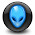# 600W Power Amplifier OCL BALAP

This amplifier OCL BALAP is class-AB with power output up to 600W using transistor final 2SC5200 2SA1943 @5pcs at 4 Ohm load with input power supply 90VDC and 300W with input supply 60VDC, below is the PCB Layout for OCL BALAP amplifier. Download the Gerber file at the end of this post.

R1/4W
10K = 6pcs
47K = 8pcs
33K = 1pcs
100R = 3pcs
470R = 3pcs
1K = 3pcs
22K = 3pcs
33R = 2pcs
330R = 2pcs
560R = 3pcs
220R = 7pcs
10R =  2pcs
2K2 = 1pcs
27K = 1pcs
270K = 1pcs

R 1/2W
4R7 = 10pcs
27K = 1pcs

R2W
11K = 2pcs
4R7 = 1pcs
0.56 = 20pcs

Trimpot 500R= 2pcs

Cap
47pF = 5pcs
10nF = 4pcs
4n7 = 2pcs
15pF = 1pcs

Elco
10uF/50V = 3pcs
220uF/35V = 1pcs
100uF/25V = 3pcs

Diode
1N4007= 1pcs
4148 = 8pcs
ZD15V = 2 pcs
ZD3.9V = 1 pcs
ZD6.2V = 1 pcs
LDR+LED
Led = 3pcs

Transistor
2N5551 = 7pcs
2N5401 = 1pcs
MPSA92 = 3pcs
BC547 = 3pcs
MJE340=2pcs
MJE350= 2pcs
BD139 = 1pcs
A1837/C4793 = 1set
2SC5200= 5pcs
2SA1943= 5pcs

IC
MC33078/NE5532 (Dual OpAmp) = 1pcs

This amplifier is recommended to use a larger heatsink so that power is not wasted a lot of heat generated by the transistor final.

Gerber FIle OCL BALAP 600W Power Amplifier

1.POR FAVOR coloque um novo link para gerber

2.Asking for PDF master the 600w ocl balap. Thanks.

3.Asking for PDF master the 600w ocl balap

4.Hello, thanks for the Gerber File for the 600W Power Amp.
I have some questions about that.
1.) with the LEDs on the PCB. Where is the anode and where is the cathode?
2.) The two big diodes on the back of the PCB, which type is that?
3.) Does LDR + LED have to be equipped? If so, where is LDR and where is LED on the board.
4.) Can you send me a schematic of the 600Watt amplifier?

5.